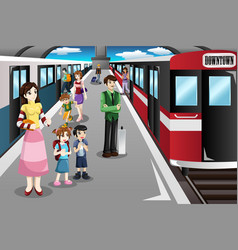# Railway ticket selling puzzle

129.3K Views

Every station on the railway system sells tickets to every other station. Some new stations were added. 46 sets of additional sets of tickets were required.

How many new stations have been added?
How many stations were there originally?2 new stations have been added & 11 stations were there originally

Explanation-
Let,
N = Original number of stations
T = # of tickets required

T= N(N-1)
T+46 = (N+X)(N+X-1)
N^2 – N + 46 = N^2+2NX+X^2-N-X
46 = X^2+2NX-X = X(X+2N-1)

Since X and N are intergers, factor 46 to get the only two integer roots 2 and 23.
2*23=X(X+2N-1) so X = 2
and 23 =(X+2N-1)  or N = 11Can you please share the calculations?

on 21st February 2020.N = Original number of stations
T = # of tickets required
T= N(N-1)
T+46 = (N+X)(N+X-1)
N^2 – N + 46 = N^2+2NX+X^2-N-X
46 = X^2+2NX-X = X(X+2N-1)
Since X and N are intergers, factor 46 to get the only two integer roots 2 and 23.
2*23=X(X+2N-1) so X = 2 and 23 =(X+2N-1)  or N = 11

on 22nd February 2020.